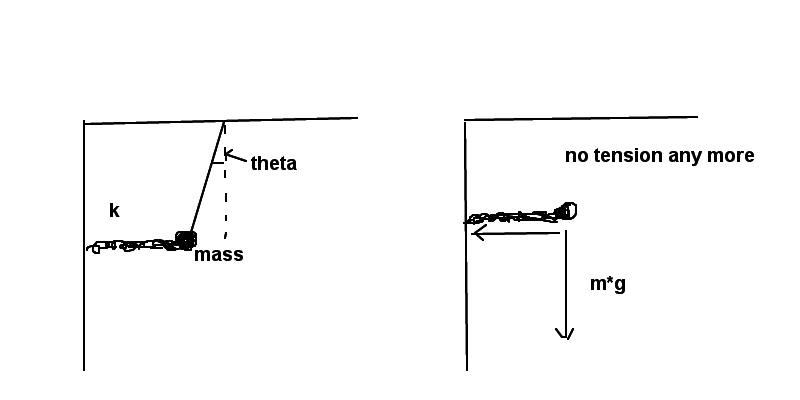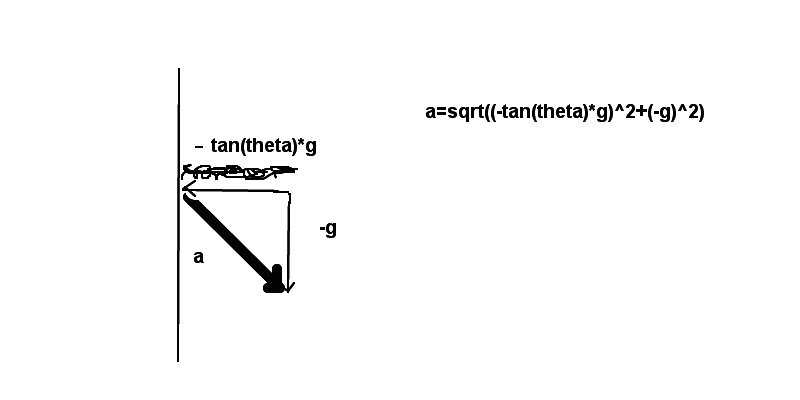# System: a rope and a spring

• Poetria
In summary, the tension in the rope is 9.8*10^-5N and the ball's acceleration immediately after the rope is cut is -2.9*10^-5 m/s^2.f

## Homework Statement

You suspend a bowling ball of mass m using a spring and a rope. The spring is horizontal and has spring constant k; the rope makes an angle θ with the vertical.

Answer all parts in terms of m, g, k, and θ.

a) What is the tension T in the rope?

b) Your arch nemesis cuts the rope. What is the acceleration of the ball immediately after the rope is cut?

## Homework Equations

Tension: (m*g)/cos(theta)

## The Attempt at a Solution

[/B]
I answered a without a problem but I have no idea what to do with b. I tried to use the vertical force when a system is in equilibrium but it doesn't work. Combinations : g*sin(theta), g*cos(theta) don't work either. I guess it is probably simple but I am at my wits' end.
The stretched distance is not given I am wondering what k is for. I could recover x by Hooke's law when the string is in a vertical position: x=-mg/k

Draw a force vector diagram and show your work, then we can see how to guide you along.

Before the rope is cut, the system is in equilibrium. Cutting the rope removes that force. How does that affect the sum of forces? Do any other forces change immediately?

(Out of interest, do you know whether you got part a) correct? If not, would you mind posting your answer?)

Thank you very much. I will draw it and post it here. I guess I have a problem with directions.
I thought I could use the formula from a somehow.I understand this is a spring and can be stretched. It is also a kind of a pendulum isn't it?

By the way I have started to learn things like that in order to understand Newton's Principia and the eighteenth-century discussions about mechanics and metaphysics (e.g. the vis viva controversy.)

I thought I could use the formula from a somehow.
You can. Just try to answer my questions in post #3.

From your free body diagram in post #5, what is the horizontal force exerted by the spring on the mass before the rope is cut? Does this force change instantly as soon as the rope is cut, or is initially still the same?

Chet

I thought this horizonal force had been initially the same. So I used the formula for the tension force.
I got:

sin(theta)*T=(sin(theta)*(m*g))/cos(theta)=tan(theta)*m*g

Of course to solve it for acceleration I divided it by the mass

a(x)=tan(theta)*g

Well, it is still wrong. :(

I thought this horizonal force had been initially the same. So I used the formula for the tension force.
I got:

sin(theta)*T=(sin(theta)*(m*g))/cos(theta)=tan(theta)*m*g

Of course to solve it for acceleration I divided it by the mass

a(x)=tan(theta)*g

Well, it is still wrong. :(
That's the correct horizontal component of the acceleration. What is the vertical component of the acceleration? From these findings, what is the resultant of the horizontal and vertical components of acceleration?

Chet

I got it. :) I messed up signs. :) Many thanks. :) :) :)